# What are some examples of direct variation in real life?Elsie

Solution- Direct variation is defined as two variables which increase or decrease at a constant rate. This constant rate is denoted by k.

The equation for direct variation can be written as,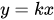Where x and y are two variables and k is a constant.

Some examples of direct variation in real life are,

1. A motor vehicle with a speed ‘x’ covers distance ‘y’ in an hour. In this case, if the speed is increased, distance covered will be more in the same amount of time.
2. If a constant ‘p’ is multiplied by numbers in increasing order, the resultant will be in increasing order.
3. A device which needs to be charged for ‘x’ hours to run for time ‘y’, in order to increase its running hours will have to be charged for more time.

Similar Problems

Question- How do you find the direct variation equation if y varies directly as x when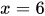,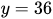?

Solution-

From the information provided in the problem,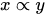The equation for direct variation is given as,Therefore,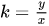On substituting the given values we get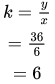Therefore the equation of direct variation will be represented as,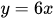Thus, the direct variation equation, if y varies directly as x, will be given as.

Question-What are some examples of inverse variation?

# Solution- Inverse function is defined as a two variables which are inversely proportional to each other. In other words, the increase in the value of one variable causes a decrease in the value of others.

Examples of such variation are,

1. A motor vehicle with a speed ‘x’ covers a particular distance in time ‘y’. In this case, if the speed is increased, the equal distance will be covered in lesser time.
2. If a constant number ‘x’ is divided by increasing values of numbers, the resultant values will be in decreasing order.
3. If a work requires 20 individual and gets completed in 10 days, the increase in manpower will lead to a decrease in time required.

### Need homework help now?

tutors online

Related Questions
##### (t) in °C of the pot of soup at time t (in The initial temperature of a pot of soup is 80°C. The temperature minutes) satisfies the differential equation dT di T-20 10 (a) Solve the differential equation, and find an explicit expression for the function (). By separating variables, integrating and manipulating arbitrary constants, . We are also told that so that and so . ...
Notes
Me

OR

Don't have an account?

Join OneClass

Access over 10 million pages of study
documents for 1.3 million courses.

Join to view

OR

By registering, I agree to the Terms and Privacy Policies
Just a few more details

So we can recommend you notes for your school.